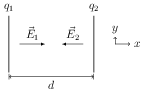# Capacitance

## Problems from IIT JEE

Problem (IIT JEE 1999): Two identical metal plates are given positive charges $q_1$ and $q_2$ $( < q_1)$ respectively. If they are now brought close together to form a parallel plate capacitor with capacitance $C$, the potential difference between them is,

1. $\frac{q_1+q_2}{2C}$
2. $\frac{q_1+q_2}{C}$
3. $\frac{q_1-q_2}{C}$
4. $\frac{q_1-q_2}{2C}$

Solution:Let $A$ be area of the plates and $d$ be separation between them. The electric field in the region between plates due to the left plate is \begin{alignat}{2} &\vec{E}_1=\frac{\sigma_1}{2\epsilon_0}\,\hat\imath=\frac{q_1}{2\epsilon_0 A}\,\hat\imath, \nonumber \end{alignat} and due to the right plate is \begin{alignat}{2} &\vec{E}_2=-\frac{\sigma_2}{2\epsilon_0}\,\hat\imath=-\frac{q_2}{2\epsilon_0 A}\,\hat\imath. \nonumber \end{alignat} The resultant field $\vec{E}$ and potential difference between two plates are, \begin{align} &\vec{E}=\vec{E}_1+\vec{E}_2=\frac{q_1-q_2}{2\epsilon_0 A}\,\hat\imath, &&V=Ed=\frac{q_1-q_2}{2(\epsilon_0 A/d)}=\frac{q_1-q_2}{2C}.\nonumber \end{align}